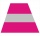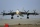# Alfa, beta, gama

In the triangle ABC is the size of the internal angle BETA 8 degrees larger than the size of the internal angle ALFA and size of the internal angle GAMA is twice the size of the angle BETA. Determine the size of the interior angles of the triangle ABC.

Result

a =  43 °
b =  51 °
c =  86 °

#### Solution:

b = 8+a
c = 2 a
a+b+c=180

a-b = -8
2a-c = 0
a+b+c = 180

a = 43
b = 51
c = 86

Calculated by our linear equations calculator.

Leave us a comment of this math problem and its solution (i.e. if it is still somewhat unclear...):Be the first to comment!#### Following knowledge from mathematics are needed to solve this word math problem:

Do you have a system of equations and looking for calculator system of linear equations? See also our trigonometric triangle calculator.

## Next similar math problems:

1. It is rectangular?Size of two of the angles in a triangle are: α=110°, β=40°. Is it a right triangle?
2. A rhombusA rhombus has sides of length 10 cm, and the angle between two adjacent sides is 76 degrees. Find the length of the longer diagonal of the rhombus.
3. The hemisphereThe hemisphere container is filled with water. What is the radius of the container when 10 liters of water pour from it when tilted 30 degrees?
4. Isosceles triangle 10In an isosceles triangle, the equal sides are 2/3 of the length of the base. Determine the measure of the base angles.
5. Hole's anglesI am trying to find an angle. The top of the hole is .625” and the bottom of the hole is .532”. The hole depth is .250” what is the angle of the hole (and what is the formula)?
6. Angles of elevationFrom points A and B on level ground, the angles of elevation of the top of a building are 25° and 37° respectively. If |AB| = 57m, calculate, to the nearest meter, the distances of the top of the building from A and B if they are both on the same side of t
7. RiverCalculate how many promiles river Dunaj average falls, if on section long 957 km flowing water from 1454 m AMSL to 101 m AMSL.
8. Trigonometric functionsIn right triangle is: ? Determine the value of s and c: ? ?
9. Is right?Is triangle with sides 51, 56 and 77 right triangle?
10. Cone A2VSurface of cone in the plane is a circular arc with central angle of 126° and area 415 cm2. Calculate the volume of a cone.
11. RiverFrom the observatory 11 m high and 24 m from the river bank, river width appears in the visual angle φ = 13°. Calculate width of the river.
12. ObserverThe observer sees straight fence 100 m long in 30° view angle. From one end of the fence is 153 m. How far is it from the another end of the fence?
13. TriangleTriangle KLM is given by plane coordinates of vertices: K[11, -10] L[10, 12] M[1, 3]. Calculate its area and itsinterior angles.
14. RightDetermine angles of the right triangle with the hypotenuse c and legs a, b, if: ?
15. PropellerThe aircraft propeller rotates at an angular speed of 200 rad/s. A) What is the speed at the tip of the propeller if its distance from the axis of rotation is 1.5 m? B) What path does the aircraft travel during one revolution of the propeller at a speed
16. HandsThe clock shows 12 hours. After how many minutes will angle between the hour and minute hand 90°? Consider the continuous movement of both hands hours.
17. ClockHow many times a day hands on a clock overlap?